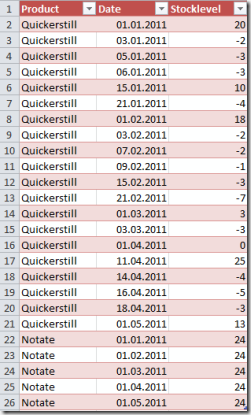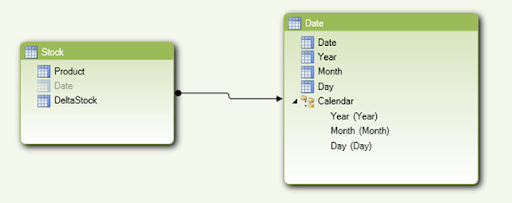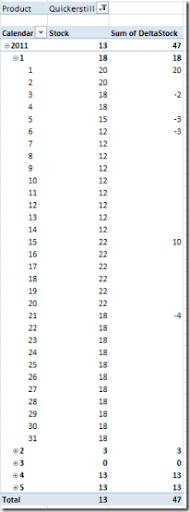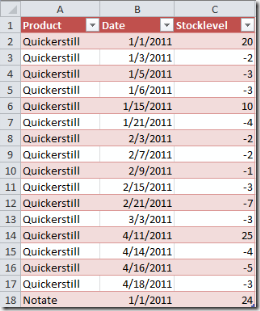## Saturday, November 19, 2011

### Stock levels as delta rows in DAX / BISM Tabular

SQL Server Denali | PowerPivot

A common way to compress large amounts of snap shot data is to store delta values instead of each snapshot value. This makes sense, if the data does not change every day. But for a good query performance you might not want to aggregate data over long periods.Therefore it makes sense to have a regular absolute snapshot value in the data and to use deltas between those snapshots. The work needed to create the periodic snapshots is usually done in the ETL process.

For my example, I’m using the following data table with absolute snapshot values as the first row of the month and deltas afterwards:For example, for our product Quickerstill we start with a stock level of 20 boxes at the beginning of January 2011. Then, at January 3, 2011 we sold two of them (-2) and again on January 5 another 3 boxes (-3).

The goal is to create a measure that gives us the current stock level at each date (also on the dates between the delta rows).

In order to do so, we need a ‘real’ date dimension, so we have a separate date table that is linked to our facts. This is how this simple model looks like:The measure DeltaStock is the original stock value from our table above (mixture of snapshots and deltas).

In order to perform the desired computation we can simply use the month-to-date formula as each day’s value computes as the sum from the first day of the month to the current day (including all deltas).

This is the simple formula we’re using to compute the stock at each day:

Stock:=TOTALMTD(SUM([DeltaStock]),'Date'[Date])

It’s amazing how simple this calculation is. Let’s take a look at the result. In order to see the effect, I added the original stock column together with the new computed column.As you can see, we now have a stock for each day that computes correctly from the mixture of snapshot and deltas values.

Of course we could also do the calculation without the absolute snapshot values in between. In this case we have to aggregate the values from the very beginning up to the current date. First let’s take a look at the source data without the absolute snapshots:The data is pretty much the same as in the first approach. Only the rows for the absolute snapshots are missing (apart from the first initial values).

In this case the calculation would look like this:

Stock:=
SumX(
DATESBETWEEN(
'Date'[Date]
, date(2000,1,1)
, lastdate('Date'[Date])
)
,calculate(
Sum('Stock'[DeltaStock])
,ALLEXCEPT('Date','Date'[Date])
)
)

The calculation is still pretty simple. However I would prefer the option with the snapshot values in between for performance reasons and because these snapshots can be easily created in ETL (if they are not delivered from the source system).

Just two more remarks before I finish this post. The first one is about the DatesBetween range in the formula above. I’m using 2000/1/1 as the start date. However if you take a look at the returned dates only the existing rows from our date table are returned if they are matching this date range. So we could also write 1900/1/1 without risking to end up with a lot of rows.

The other remark is about the future time. Since our calculation takes the last value as the value for all the future, you will find values for all entries of the date dimension. This might not be wanted. In this case you can wrap the calculation from above inside an if statement to check the date:

StockClipped:=
if(
firstdate('Date'[Date])>Now()
, Blank()
,SumX(
DATESBETWEEN(
'Date'[Date]
, date(2000,1,1)
, lastdate('Date'[Date])
)
,calculate(
Sum('Stock'[DeltaStock])
,ALLEXCEPT('Date','Date'[Date])
)
)
)

With this modification (which also works with the formula for the absolute intermediate snapshots from above) values are only shown for periods that are over or have at least started. So the formula would return a value for the full year 2011 once the year has started. If you only want to see values for periods that have ended, you can replace the function ‘firstdate’ with a ‘lastdate’.